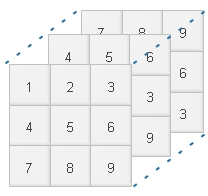# tensorflow Basics

## What is Tensor

In simple language we can call as multi dimensional array. Let us try to understand different dimensionsZero dimension : It is a single point or a scalar value
One dimension : It is a line , it consist of zero dimension points
Two dimensions : It is a matrix ,
N dimensions : Tensor

## What is graph and what is session

Tensors flow across a graph consist of operations. Here tensors flows through each node or operation under a session. The graph consist of nodes or operations and tensors are our inputs or resultants out of the nodes.

Each session is a process flow across the graph using nodes and tensors.
Having said all these complex words to understand let us first start with some examples.

Importing library
``import tensorflow as tf``
Creating graph
``````import tensorflow as tf
my_graph = tf.Graph()
with my_graph.as_default():
a = tf.constant(, name = 'my_const_a')``````
Here we have added one constant. Now we will create session and see the result.
``````import tensorflow as tf
my_graph = tf.Graph()
with my_graph.as_default():
a = tf.constant(, name = 'my_const_a')
my_sess = tf.Session(graph = my_graph)
result = my_sess.run(a)
print(result)
sess.close()``````
Output
````

``````import tensorflow as tf
my_graph = tf.Graph()
with my_graph.as_default():
a = tf.constant(, name = 'my_const_a')
b = tf.constant(, name = 'my_const_b')
sess = tf.Session(graph = my_graph)
result = sess.run(c)
print(result)
sess.close()``````
Output
````
We will get shape of different dimensions
``````import tensorflow as tf
my_graph = tf.Graph()
with my_graph.as_default():
my_scalar = tf.constant(5)
my_vector = tf.constant([1,2,3])
my_matrix = tf.constant([[1,2,3],[4,5,6],[7,8,9]])
my_tensor = tf.constant( [ [[1,2,3],[4,5,6],[7,8,9]] ,
[[4,5,6],[1,2,3],[7,8,9]] , [[7,8,9],[4,5,6],[1,2,3]] ] )

print(my_scalar.shape)
print(my_vector.shape)
print(my_matrix.shape)
print(my_tensor.shape)``````
Output
``````()
(3,)
(3, 3)
(3, 3, 3)``````
Now let us multiply two matrix by using matmul()
``````import tensorflow as tf
my_graph = tf.Graph()
with my_graph.as_default():
my_matrix1=tf.constant([[1,2,3],[4,5,6],[7,8,9]])
my_matrix2=tf.constant([[11,12,13],[14,15,16],[17,18,19]])
my_result=tf.matmul(my_matrix1,my_matrix2)
with tf.Session(graph = my_graph) as my_sess:
result=my_sess.run(my_result)
print(result)``````
Output
``````[[ 90  96 102]
[216 231 246]
[342 366 390]]``````

Subscribe to our YouTube Channel here

## Subscribe

* indicates required
Subscribe to plus2netplus2net.com